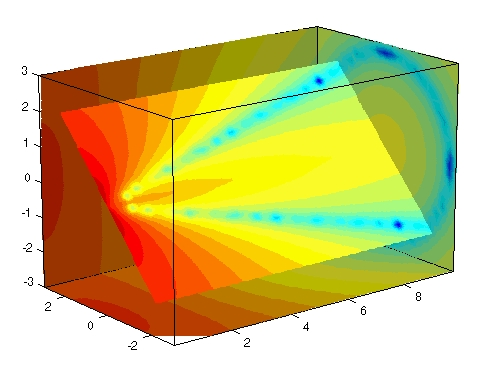3-D VisualizationExample -- Slicing Fluid Flow Data

This example slices through a volume generated by the `flow` M-file.

1. Investigate the Data

Generate the volume data with the command

• ```[x,y,z,v] = flow;
```

Determine the range of the volume by finding the minimum and maximum of the coordinate data.

• ```xmin = min(x(:));
ymin = min(y(:));
zmin = min(z(:));

xmax = max(x(:));
ymax = max(y(:));
zmax = max(z(:));
```

2. Create a Slice Plane at an Angle to the X-Axes

To create a slice plane that does not lie in an axes plane, first define a surface and rotate it to the desired orientation. This example uses a surface that has the same x- and y- coordinates as the volume.

• ```hslice = surf(linspace(xmin,xmax,100),...
linspace(ymin,ymax,100),...
zeros(100));
```

Rotate the surface by -45 degrees about the x-axis and save the surface `XData`, `YData`, and `ZData` to define the slice plane; then delete the surface.

• ```rotate(hslice,[-1,0,0],-45)
xd = get(hslice,'XData');
yd = get(hslice,'YData');
zd = get(hslice,'ZData');
delete(hslice)
```

3. Draw the Slice Planes

Draw the rotated slice plane, setting the `FaceColor` to `interp` so that it is colored by the figure colormap, and set the `EdgeColor` to `none`. Increase the `DiffuseStrength` to `.8` to make this plane shine more brightly after adding a light source.

• ```h = slice(x,y,z,v,xd,yd,zd);
set(h,'FaceColor','interp',...
'EdgeColor','none',...
'DiffuseStrength',.8)
```

Set `hold` to on and add three more orthogonal slice planes at `xmax`, `ymax`, and `zmin` to provide a context for the first plane, which slices through the volume at an angle.

• ```hold on
hx = slice(x,y,z,v,xmax,[],[]);
set(hx,'FaceColor','interp','EdgeColor','none')

hy = slice(x,y,z,v,[],ymax,[]);
set(hy,'FaceColor','interp','EdgeColor','none')

hz = slice(x,y,z,v,[],[],zmin);
set(hz,'FaceColor','interp','EdgeColor','none')
```

4. Define the View

To display the volume in correct proportions, set the data aspect ratio to `[1,1,1]` (`daspect`). Adjust the axis to fit tightly around the volume (`axis`) and turn on the `box` to provide a sense of a 3-D object. The orientation of the axes can be selected initially using `rotate3d` to determine the best `view`.

Zooming in on the scene provides a larger view of the volume (`camzoom`). Selecting a projection type of `perspective` gives the rectangular solid more natural proportions than the default orthographic projection (`camproj`).

• ```daspect([1,1,1])
axis tight
box on
view(-38.5,16)
camzoom(1.4)
camproj perspective
```

5. Add Lighting and Specify Colors

Adding a light to the scene makes the boundaries between the four slice planes more obvious because each plane forms a different angle with the light source (`lightangle`). Selecting a colormap with only 24 colors (the default is 64) creates visible gradations that help indicate the variation within the volume.

• ```lightangle(-45,45)
colormap (jet(24))
set(gcf,'Renderer','zbuffer')```

The Modifying the Color Mapping section shows how to modify how the data is mapped to color.Exploring Volumes with Slice Planes Modifying the Color Mapping© 1994-2005 The MathWorks, Inc.